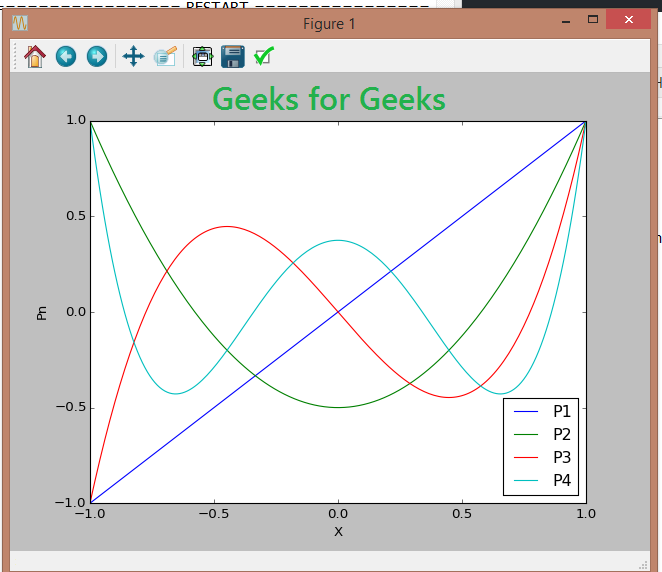# Python – Legendre polynomials using Recursion relation

Legendre polynomials are a type of orthogonal polynomials which occur often in science and engineering. Hence, their generation is crucial to those fields. There are different ways to evaluate a Legendre polynomial, using generating functions, Rodrigues formula, recurrence relation, Gram-Schmidt orthogonalization etc. One of the easiest and also
one of the most accurate, method is using recurrence relation.

Here we use Bonnet’s recurrence relation of legendre polynomials, i.e, –It can be implemented using Python by proceeding as follows-

We define Legendre polynomials as a function named P(n, x), where n is called the order of the polynomial and x is the point of evaluation. The base cases are if n is 0, then The value of the polynomial is always 1, and it is x when order is 1. These are the seed values required for the recurrence relation.
For other values of n, the function is recursively defined, directly from the Bonnet’s recurrence. Thus, P(n, x) returns values of the Legendre polynomial, by recursion method (A function effectively defined with other base cases of the same function itself.)

Below is the Python implementation –

 `# Legendre polynomial ` `def` `P(n, x):  ` `    ``if``(n ``=``=` `0``): ` `        ``return` `1` `# P0 = 1 ` `    ``elif``(n ``=``=` `1``): ` `        ``return` `x ``# P1 = x ` `    ``else``: ` `        ``return` `(((``2` `*` `n)``-``1``)``*``x ``*` `P(n``-``1``, x)``-``(n``-``1``)``*``P(n``-``2``, x))``/``float``(n) ` ` `  `# Suppose, we want to find the value of  ` `# 3rd order legendre polynomial at x = 5 ` `# We can display the value by-- ` ` `  `# Driver program ` `n ``=` `3` `X ``=` `5` `print``(``"The value of the polynomial at given point is:"``, P(n, X)) `

Output:

```The value of the polynomial at given point is: 305.0
```

We can now also plot the Legendre polynomials (say from 1st order to 4th order) using matplotlib.

 `import` `matplotlib ` ` `  `# This is for use in webbrowser, can be ignored. ` `matplotlib.use(``'Agg'``)  ` ` `  `import` `matplotlib.pyplot as plt ` `import` `numpy as np ` ` `  `# Creating an array of x values ` `x ``=` `np.linspace(``-``1``, ``1``, ``200``)  ` ` `  `# for which polynomial values are evaluated and plotted ` `for` `i ``in` `range``(``1``, ``5``): ` ` `  `    ``# Labelling according to order ` `    ``plt.plot(x, P(i, x), label ``=``"P"``+``str``(i))  ` ` `  `plt.legend(loc ``=``"best"``) ` `plt.xlabel(``"X"``) ` `plt.ylabel(``"Pn"``) ` `plt.show() `Don’t stop now and take your learning to the next level. Learn all the important concepts of Data Structures and Algorithms with the help of the most trusted course: DSA Self Paced. Become industry ready at a student-friendly price.

My Personal Notes arrow_drop_upCheck out this Author's contributed articles.

If you like GeeksforGeeks and would like to contribute, you can also write an article using contribute.geeksforgeeks.org or mail your article to contribute@geeksforgeeks.org. See your article appearing on the GeeksforGeeks main page and help other Geeks.

Please Improve this article if you find anything incorrect by clicking on the "Improve Article" button below.